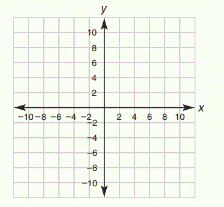Chapter 10.CT, Problem 6CT### Elementary Geometry for College St...

6th Edition
Daniel C. Alexander + 1 other
ISBN: 9781285195698

#### Solutions

Chapter
Section### Elementary Geometry for College St...

6th Edition
Daniel C. Alexander + 1 other
ISBN: 9781285195698
Textbook Problem
1 views

# Using the table from Exercise 5, sketch the graph of 2 x + 3 y = 12 .To determine

To draw:

The graph of the equation 2x+3y=12

Explanation

To draw a graph for a equation,

First we need to find the x-intercept and y-intercept.

For finding the x-intercept, make y as 0 and solve for x in the given equation.

For finding the y-intercept, make x as 0 and solve for y in the given equation.

Substitute x=0 in the given equation 2x+3y=12,

20+3y=12

On simplifying,

3y=12

The coefficient of y is 3, thus divide both sides by 3.

3y3=123

y=4

Thus the first point is 0, 4.

Substitute x=3 in the given equation 2x+3y=12,

23+3y=12

On simplifying,

6+3y=12

3y=12-6

3y=6

The coefficient of y is 3, thus divide both sides by 3.

3y3=63

y=2

Thus the second point is 3, 2.

Substitute y=4 in the given equation 2x+3y=12,

2x+34=12

On simplifying,

2x+12=12

2x=12-12

2x=0

x=0

Thus the third point is 0, 4.

Substitute x=9 in the given equation 2x+3y=12,

29+3y=12

On simplifying,

18+3y=12

3y=12-18

3y=-6

The coefficient of y is 3, thus divide both sides by 3

### Still sussing out bartleby?

Check out a sample textbook solution.

See a sample solution

#### The Solution to Your Study Problems

Bartleby provides explanations to thousands of textbook problems written by our experts, many with advanced degrees!

Get Started

#### (t+6)(60)(60t+180)(t+6)2

Applied Calculus for the Managerial, Life, and Social Sciences: A Brief Approach

#### Compute P7,7.

Understanding Basic Statistics

#### Define fraud and explain the safeguards that exist to prevent it.

Research Methods for the Behavioral Sciences (MindTap Course List)